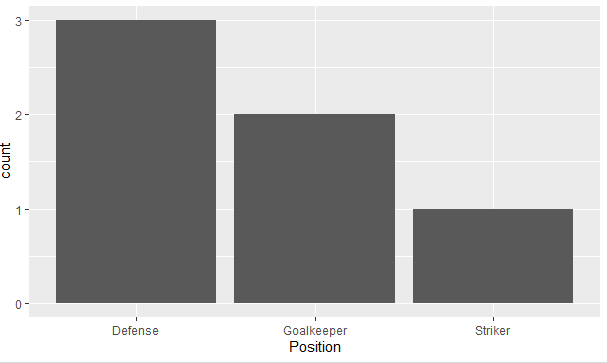2 views

I am trying to make a bar graph where the largest bar would be nearest to the y-axis and the shortest bar would be furthest. So this is kind of like the Table I have

Name   Position

1   James  Goalkeeper

2   Frank  Goalkeeper

3   Jean   Defense

4   Steve  Defense

5   John   Defense

6   Tim    Striker

So I am trying to build a bar graph that would show the number of players according to the position

p <- ggplot(theTable, aes(x = Position)) + geom_bar(binwidth = 1)

but the graph shows the goalkeeper bar first then the defense, and finally the striker one. I would want the graph to be ordered so that the defense bar is closest to the y-axis, the goalkeeper one, and finally the striker one. Thanks

by

To order bars in a ggplot2 bar graph, you need to factor the variable and set the levels of the variable that you are plotting.

To create the data frame:

theTable <- data.frame(Name = c("James","Frank","Jean","Steve","John","Tim"),

Position  = c("Goalkeeper","Goalkeeper","Defense","Defense","Defense","Striker"), stringsAsFactors = FALSE)

To factor and sort levels for ‘position’:

theTable\$Position <- factor(theTable\$Position, levels = names(sort(table(Position), decreasing=TRUE)))

To plot the data:

ggplot(theTable,aes(x=Position))+geom_bar()

Output: